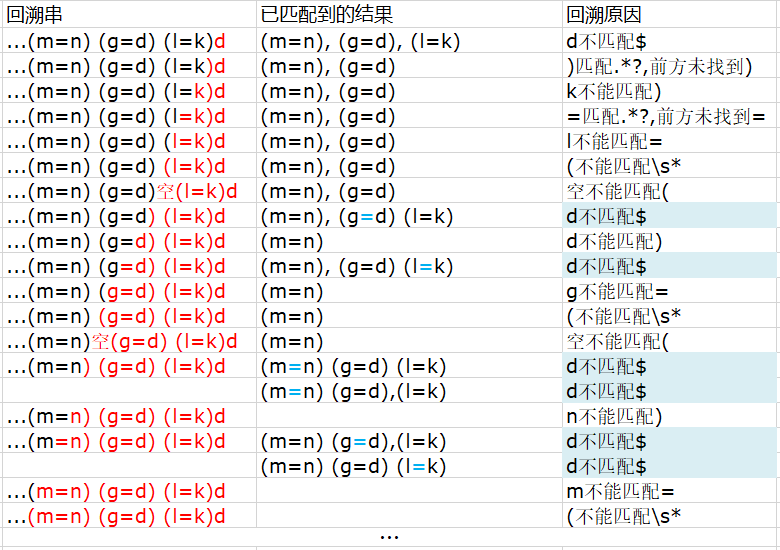# Js正则表达式——引用匹配组Javascript 同时被 2 个专栏收录11 篇文章 0 订阅

Javascript的正则支持组引用，即在正则式中使用已经匹配到的子串。什么意思呢？先看个问题：我要一个正则，匹配2080-02-262080.02.26这两个时间格式，怎么写呢？/^\d+[.\-]\d+[.\-]\d+$/显然是不行的，因为它也能匹配2080-02.26,2080.02-26，这时我们就可以使用正则提供的引用功能。 ##### 组引用 它的写法是\n，看起来怎么跟个换行符一样？其实n指的是已经匹配到的子串的序号，而不是字母'n'，像上面那个问题可以写成/^\d+([.\-])\d+\1\d+$/，再来看看效果：

/^\d+([.\-])\d+\1\d+$/.test('2019-08-19'); // true /^\d+([.\-])\d+\1\d+$/.test('2019.08.19'); // true
/^\d+([.\-])\d+\1\d+$/.test('2019-08.19'); // false /^\d+([.\-])\d+\1\d+$/.test('2019.08-19'); // false


MDN上有一个类似的例子：/(?:\d{3}|$$\d{3}$$)([-\/\.])\d{3}\1\d{4}/，匹配(020)-885-6652,032/565/9656,365.356.3333这种电话号码格式，其实原理也是一样，电话号之间的分隔只能同时是./或者-

##### 消除回溯

var ss = '(a=b) (a=c) (b=c) (d=f) (g=i) (h=i) (q=o) (e=0) (p=r) (s=3) (x=3) (h=e) (5=d) (m=n) (g=d) (l=k)';

console.time('reg5');
ss.match(reg5);
console.timeEnd('reg5'); // reg5: 1.642822265625ms


var reg5 = /(\s*$$.*?=.*?$$)+$/; var ss = '(a=b) (a=c) (b=c) (d=f) (g=i) (h=i) (q=o) (e=0) (p=r) (s=3) (x=3) (h=e) (5=d) (m=n) (g=d) (l=k)d'; console.time('reg5'); ss.match(reg5); console.timeEnd('reg5'); // reg5: 1391.31298828125ms  这个正则耗时1391ms，如果待匹配的字符串变长，这个时间可能更长甚至直接卡死，使用中的CPU核心占用率达到100% 为什么会这样呢？来分析一下正则的回溯过程：我们只分析了后三个匹配到的子串，这个回溯会一直进行到字符串开头，其中还包含了一些子回溯（青色背景的单元格），所以这个正则表达式的执行效率相当低，很容易造成卡死，这种现象叫做“灾难性回溯”(catastrophic backtracking)。 其实按照正常人的思维，这个字符串最后一位不是)，而正则要求最后一位必是)，显然它就不能匹配上该字符串。不管你怎么回溯，都改变不了最后一位肯定不能匹配上的事实。很明显，这里的回溯做了很多无用功，甚至引发了灾难级的重复计算。 那怎么在代码里解决这个问题呢？很多编程语言如Ruby，Java，Perl的正则都提供了一个叫做“原子组”(atomic grouping)的东西，原子组在成功匹配一次之后，就抛弃所有内存中的其他可能匹配。也就是说，它成功匹配到一次之后，不会再尝试其他可能值。比如说一个a(?>bc|b)c的正则表达式，可以匹配'abcc'但是不能匹配'abc'，因为'abc'先消耗了原子组里的bc，然后发生了回溯，但是此时原子组的值就是'bc'，它再也无法匹配到原子组里面的b了。 遗憾的是，Javascript的正则系统并不支持原子组，但是可以通过组引用解决类似问题。 我们把正则写成这种形式/(?=(\s*$$.*?=.*?$$)+)\1$/，与组相关的正则式被写在了一个前环视中（环视是ES6新增的正则功能，不清楚的话可以看前一篇博客），然后在环视匹配后使用\1消耗掉匹配到的组

var reg6 = /(?=(\s*$$.*?=.*?$$)+)\1$/; var ss = '(a=b) (a=c) (b=c) (d=f) (g=i) (h=i) (q=o) (e=0) (p=r) (s=3) (x=3) (h=e) (5=d) (m=n) (g=d) (l=k)'; console.time('reg6'); console.log(ss.match(reg6)); // [" (l=k)", " (l=k)", index: 89, ... console.timeEnd('reg6'); // reg6: 1.5791015625ms  功能正常，可以匹配成功，匹配组对应的是' (l=k)'，即最后一个匹配的子串。 再来看匹配失败的情况： var reg6 = /(?=(\s*$$.*?=.*?$$)+)\1$/;
var ss = '(a=b) (a=c) (b=c) (d=f) (g=i) (h=i) (q=o) (e=0) (p=r) (s=3) (x=3) (h=e) (5=d) (m=n) (g=d) (l=k)d';
console.time('reg6');
console.log(ss.match(reg6)); // null
console.timeEnd('reg6'); // reg6: 2.432861328125ms


##### 参考资料：

. js无限回溯问题
. 原子组
. 向前看的方法解决回溯

01-06447
08-2417207-19905
11-1870
07-283747
09-018482
04-251万+
09-111万+
09-174039
11-111521
04-261087
03-251529
11-241万+
11-12109
03-28412点击重新获取扫码支付余额充值